Question

# The mean incubation time of fertilized eggs is 19 days. Suppose the incubation times are approximately...

The mean incubation time of fertilized eggs is 19 days. Suppose the incubation times are approximately normally distributed with a standard deviation of 1 day. ​(a) Determine the 10th percentile for incubation times. ​(b) Determine the incubation times that make up the middle 97​%.

TOPIC:Use of the Normal distribution.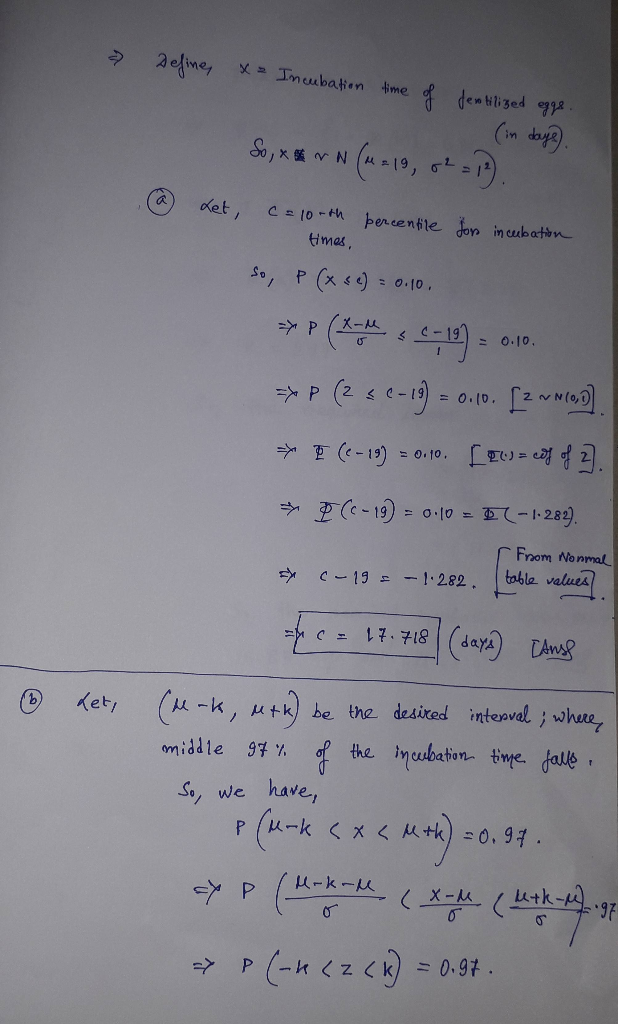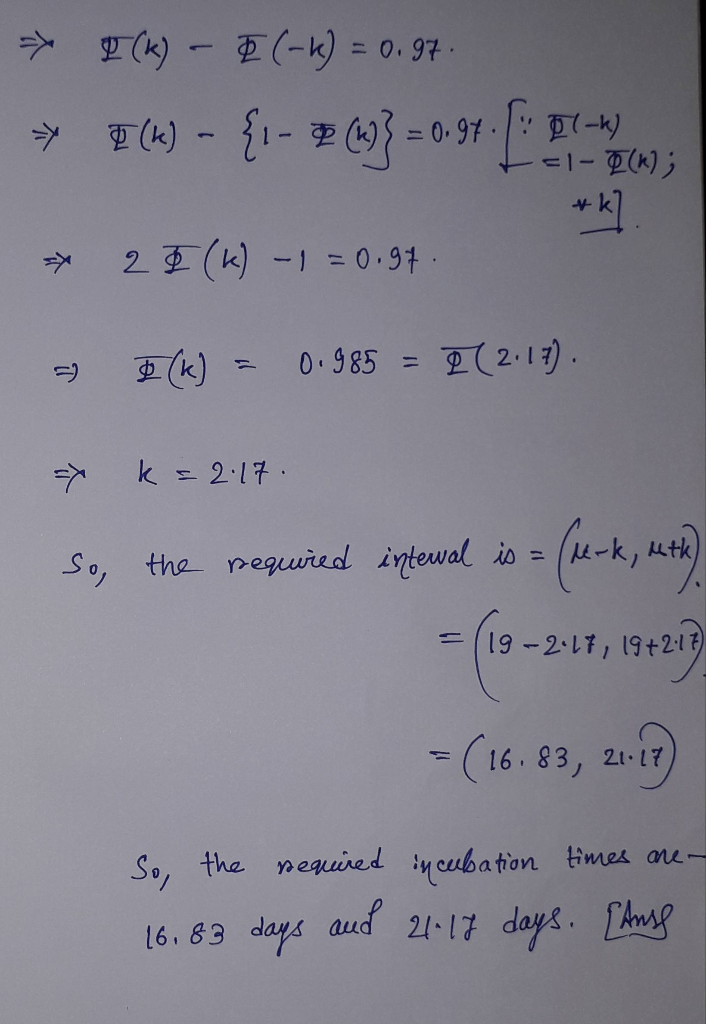#### Earn Coins

Coins can be redeemed for fabulous gifts.

Similar Homework Help Questions
• ### The mean incubation time of fertilized eggs is 21 days. Suppose the incubation times are approximately...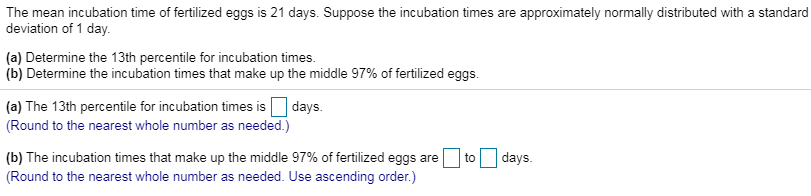The mean incubation time of fertilized eggs is 21 days. Suppose the incubation times are approximately normally distributed with a standard deviation of 1 day. (a) Determine the 13th percentile for incubation times. (b) Determine the incubation times that make up the middle 97% of fertilized eggs. (a) The 13th percentile for incubation times is days. (Round to the nearest whole number as needed.) to days. (b) The incubation times that make up the middle 97% of fertilized eggs are...

• ### The mean incubation time of fertilized eggs is 22 days. Suppose the incubation times are approximately...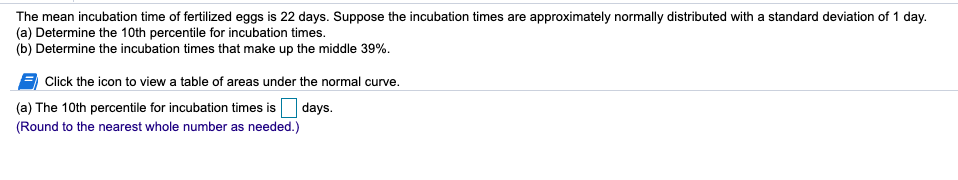The mean incubation time of fertilized eggs is 22 days. Suppose the incubation times are approximately normally distributed with a standard deviation of 1 day. (a) Determine the 10th percentile for incubation times. (b) Determine the incubation times that make up the middle 39%. Click the icon to view a table of areas under the normal curve. (a) The 10th percentile for incubation times is days. (Round to the nearest whole number as needed.)

• ### The mean incubation time of fertilized eggs is 23 days. Suppose the incubation times are approximately...

The mean incubation time of fertilized eggs is 23 days. Suppose the incubation times are approximately normally distributed with a standard deviation of 1 day. ​(a) Determine the 14th percentile for incubation times. ​(b) Determine the incubation times that make up the middle 95​%. LOADING... Click the icon to view a table of areas under the normal curve. ​(a) The 14th percentile for incubation times is nothing days. ​(Round to the nearest whole number as​ needed.)

• ### The mean incubation time of fertilized eggs is 23 days, Suppose the incubation times are approximately...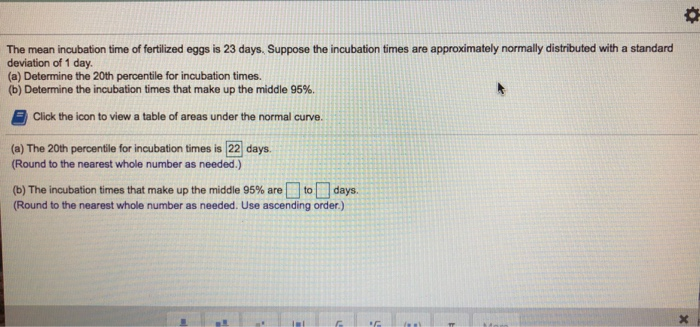The mean incubation time of fertilized eggs is 23 days, Suppose the incubation times are approximately normally distributed with a standard deviation of 1 day (a) Determine the 20th percentile for incubation times. (b) Determine the incubation times that make up the middle 95%. Click the icon to view a table of areas under the normal curve. (a) The 20th percentle for incubation times is 22 days. (Round to the nearest whole number as needed.) (b) The incubation times that...

• ### The mean incubation time of fertilized eggs is 23 days

The mean incubation time of fertilized eggs is 23 days. Suppose the incubation times are approximately normally distributed with a standard deviation of 1 day. (a) Determine the 15th percentile for incubation times. (b) Determine the incubation times that make up the middle 95% of fertilized eggs. (a) The 15th percentile for incubation times is _______  days. (Round to the nearest whole number as needed) (b) The incubation times that make up the middle 95% of fertilized eggs are _______  to _______  days. (Round...

• ### standard deviation of 1 day. The mean incubation time of fertilized eggs is 21 days. Suppose...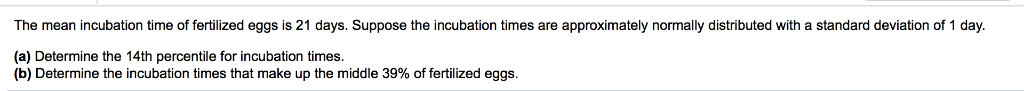standard deviation of 1 day. The mean incubation time of fertilized eggs is 21 days. Suppose the incubation times are approximately normally distributed with (a) Determine the 14th percentile for incubation times. (b) Determine the incubation times that make up the middle 39% of fertilized eggs. The time required for an automotive center to complete an oil change service on an automobile approximately follows a normal distribution, with a mean of 19 minutes standard deviation of 3 minutes. and (a)...

• ### This Question: 6 pts 7 9 of 15 (10 complete) This Test: 100 pts possible E...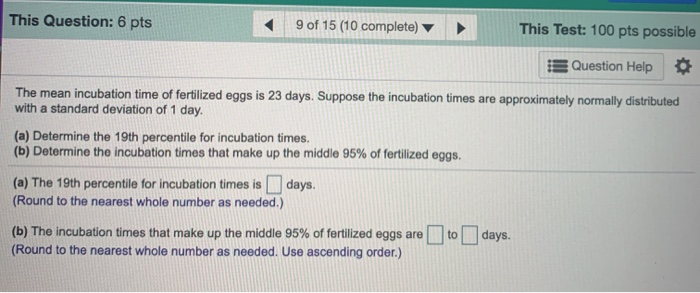This Question: 6 pts 7 9 of 15 (10 complete) This Test: 100 pts possible E Question Help The mean incubation time of fertilized eggs is 23 days. Suppose the incubation times are approximately normally distributed with a standard deviation of 1 day. (a) Determine the 19th percentile for incubation times. (b) Determine the incubation times that make up the middle 95% of fertilized eggs. (a) The 19th percentile for incubation times is days. (Round to the nearest whole number...

• ### The time required for an automotive center to complete the service oil change service on an automobile approximately follows a normal distribution

1.The time required for an automotive center to complete the service oil change service on an automobile approximately follows a normal distribution, with a mean 19 minutes and a standard deviation of 3 minutes.  a. The automotive center guarantees customers that the service will take no longer than 20 minutes. If it does take ionger, the customer will receive the service for half-price. What percent of customers recelve the service for half-price? b. If the automotive center does not want to give the...

• ### The mean incubation time for a type of fertilized egg kept at 100.2​°F is 23 days....

The mean incubation time for a type of fertilized egg kept at 100.2​°F is 23 days. Suppose that the incubation times are approximately normally distributed with a standard deviation of 1 day. ​(a) What is the probability that a randomly selected fertilized egg hatches in less than 22 ​days? ​(b) What is the probability that a randomly selected fertilized egg hatches between 21 and 23 ​days? ​(c) What is the probability that a randomly selected fertilized egg takes over 24...

• ### 7.2 Applications of the Normal Distribution 7.2.RA-3 0 of 1 Point Question Help The mean incubation...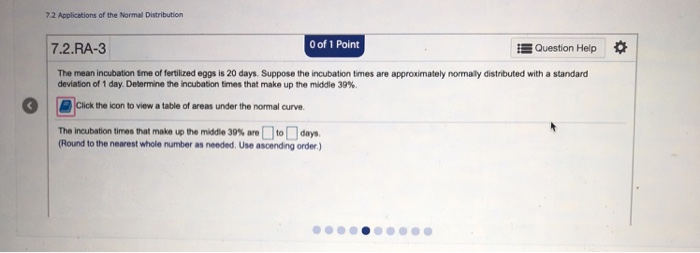7.2 Applications of the Normal Distribution 7.2.RA-3 0 of 1 Point Question Help The mean incubation time of fertilized eggs is 20 days. Suppose the incubation times are approximately normally distributed with a standard deviation of 1 day. Determine the incubation times that make up the middle 39% Click the icon to view a table of areas under the normal curve. The incubation times that make up the middle 30% arou today (Round to the nearest whole number as needed....